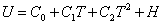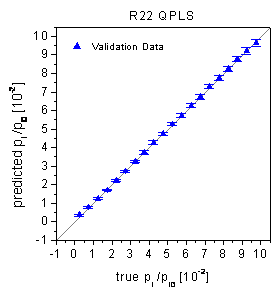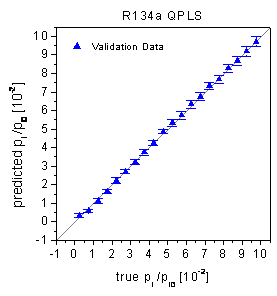Home News About Me Ph. D. Thesis Abstract Table of Contents 1. Introduction 2. Theory  Fundamentals of the Multivariate Data Analysis 3. Theory  Quantification of the Refrigerants R22 and R134a: Part I 4. Experiments, Setups and Data Sets 5. Results  Kinetic Measurements 6. Results  Multivariate Calibrations 6.1. PLS Calibration 6.2. Box-Cox Transformation + PLS 6.3. INLR 6.4. QPLS 6.5. CART 6.6. Model Trees 6.7. MARS 6.8. Neural Networks 6.9. PCA-NN 6.10. Neural Networks and Pruning 6.11. Conclusions 7. Results  Genetic Algorithm Framework 8. Results  Growing Neural Network Framework 9. Results  All Data Sets 10. Results  Various Aspects of the Frameworks and Measurements 11. Summary and Outlook 12. References 13. Acknowledgements Publications Research Tutorials Downloads and Links Contact Search Site Map Print this Page## 6.4.   QPLS

In the field of QSAR research, a polynomial expansion of the inner relationship, which is linear in the original PLS, has become popular to model nonlinear relationships ,-. If the polynomial terms are of the second order, this approach is also known as QPLS (quadratic PLS). Instead of the linear relationship between the score matrixes U and T, following poly­nomial expression is used:(30)

The coefficients C0, C1 and C2 are determined by the least squares method in an iterative procedure similar to the PLS. For the calibration data, QPLS models were built with an optimal number of principal components determined by a minimum crossvalidation error of the calibration data. For R22 the optimal model with 2 principal components predicted the calibration data with a rel. RMSE of 2.31% and the validation data with a rel. RMSE of 2.41%. For R134a the optimal model with 4 principal components predicted the calibration data with a rel. RMSE of 3.87% and the validation data with a rel. RMSE of 3.92%. The sensibly low number of principal components is "rewarded" by practically identical validation errors and calibration errors. Both, the Wald-Wolfowitz Runs test and the Durbin-Watson Statistic cannot find a significant non-randomness in the prediction of the validation data. In combination with the true-predicted plots (figure 36) and the low errors of prediction, it is obvious that among the different PLS approaches the QPLS can deal best with the nonlinear data set. In the next sections, several non-PLS methods, which are also know to be able to account for nonlinearities, are applied to the calibration and validation data set.figure 36:  True-predicted plots of the QPLS for the validation data.

 Page 93 © Frank Dieterle, 03.03.2019